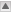## Meaning of IsEm bits in 12.5.0:

Under Construction!

Note: This refers to release 12.5.0 but only if I've understood how the tagcollector and so forth works.

• The ElectroMagnetic object identifier flag IsEM contains a bit pattern with the results of various calorimeter and tracking criteria applied in the ID. Post 12.04 (or thereabouts) the IsEm flag gets to carry more information. This description refers to that more detailed version. However, As far as I can see, the main change from 12.0.4 has been simply to assign separate bits to some of the information previously combined into fewer single bits. Thus the algorithms used and the set of criteria to be satisfied in order for IsEm==0 is unchanged.

• The bit pattern is set in: Reconstruction/egammaRec/EMPIDBuilder.cxx

#### Definition of the bits

(See egammaPIDdefs.h)

• NameBit
Cluster based egamma
ClusterEtaRange 0
ClusterMiddleEnergy 4
ClusterMiddleEratio33 6
ClusterMiddleEratio37 5
ClusterMiddleWidth 7
ClusterStripsDeltaE 10
ClusterStripsDeltaEmax2 9
ClusterStripsEratio 8
ClusterStripsFracm 12
ClusterStripsWeta1c 13
ClusterStripsWtot 11
Track based egamma
TrackA0 19
TrackBlayer 16
TrackMatchEoverP 22
TrackMatchEta 20
TrackMatchPhi 21
TrackPixel 17
TrackSi 18
TrackTRThits 23
TrackTRTratio 24

The following predefined (in egammaPIDdefs.h) bit-masks are available:
• ALL=0xFFFFFFFF;
• CALO=0xFFFF;
• CALOSTRIPS=0xFF00;
• CALOMIDDLE=0xF0;
• TRACKING =0xF0000;
• TRACKMATCH =0x700000;
• ALLNOTRT=0x7FFFFF;

• (IsEm & MASK)==0 => uses only the MASKed info and ignores the rest.
• (IsEm & ~MASK) ==0 => uses all of IsEM except the MASKed info.

#### How the bits are set:

The standard egamma analysis does the following:
• Checks for a matching track using EMTrackMatch
• If there is a matching track:
• treat as electron and apply one set of calorimeter requirements
• apply track requirements
• If no matching track:
• treat as photon and apply different set of calorimeter requirements (see below)

• IsEM flag is initialized to 0

Bits 0-13 are to do with calorimeter information. Here's how they are set in the 'electron' case:

• Bit 0: eta range cut. Bit set if abs(eta in 2nd sampling layer) > 2.47 or <=0.
• Bit 1: eta-bin-dependent cut on Hadronic leakage:
• The leakage is defined as (Et in 1st hadronic sampling layer)/(Cluster Et) (or 0 if Cluster energy is zero).
• In eta bin 0 and if Low Lumi: Bit set if: leakage >3.5*(0.01046-0.0121*eta2+0.002*eta2*eta2)
• other eta bins: Bit set if leakage > m_CutHadLeakage[ibin] where ibin depends on eta and Et.

• Bits 4-7 : eta- and Et- bin-dependent cut on energy in 2nd sampling layer.
• Bit 4: 2nd sampling layer e277 < 0
• Bit 5: e237/e277 < m_CutReta37[ibin]
• Bit 6: left unset for electron case
• Bit 7: weta2c>m_CutWeta2c[ibin] *where e237, e277 are energies in 3x7 and 7x7 cell arrays respectively in the 2nd sampling layer and weta2c is the corrected width in 3x5 cells of the 2nd sampling layer.

• Bits 8-13: eta bin dependent cuts on 1st sampling layer. The criteria are checked only if:
• fraction of energy found in 1st EM sampling layer > m_CutF1_electrons
• ...and eta2 < 2.37
• ...and eta2 < 1.37 or eta2 > 1.52 (i.e. not in crack)
• If so then...
• Bit 9: emax2>0. and deltaemax2>=m_CutDeltaEmax2_electrons[ibin]
• Bit 10: emax2>0. and (emax2-emin)>=m_CutDeltaE_electrons[ibin]
• Bit 11: wtot>=m_CutWtot_electrons[ibin]
• Bit 12: fracm >= m_CutFracm_electrons[ibin]
• Bit 13: weta1c >= m_CutWeta1c[ibin] *Where:
• emax2 is the 2nd maximum in strips (see PidElectronEvent/EMShower.h)
• deltaemax2 is a luminosity dependent variable:
• fracm is energy outside core (E(+-7)-E(+-3))/E(+-7) *
```           if (m_LumiLevel == "LowLumi") {
deltaemax2 = emax2/(1000.+0.009*et_37);
}
if (m_LumiLevel == "HighLumi") {
deltaemax2 = emax2/(1000.+0.0049*et_37);
}
```
• emin is the value of the energy minimum between the maxima emax 1 & emax2 (see PidElectronEvent/EMShower.h)
• wtot is the shower total width in em sampling 1 in 20 strips (see PidElectronEvent/EMShower.h)

For photons the selection differs slightly:
• Bit 0 set if eta2>2.37
• this is based on eta binning defined in: m_CutBinEta_photons[]

• Bits 4-7 : eta and Et bin dependent cut on energy in 2nd sampling layer.
• Bit 4: 2nd sampling layer e277 < 0.1
• Bit 5: e237/e277 < m_ratio1_photons[ibin_combined]
• Bit 6: e233/2237 < m_ratio2_photons[ibin_combined]
• Bit 7: weta2c>m_weta2_photons[ibin_combined]
• The binning here is in eta and cluster Et
• Bits 8-13: cuts on the strip
• Bit 8: fraction of energy in 1st sampling layer < 0.005
• Bit 9: deltaemax2 > m_emax2r_photons[bin]
• Bit 10: deltae > m_deltae_photons[bin]
• Bit 11: wtot > m_wtot_photons[bin]
• Bit 12: fracm > m_fracm_photons[bin]
• Bit 13: weta1c > m_w1_photons[bin]
• See electron cuts for meanings of the variables.
• bins are in eta and Et

In the case where there is a Matching Track, the following track quality cuts are applied:

• Bits 16-17 set if too few b-layer, pixel, or Si hits or if the impact parameter is too high
• Bit 16: nBL<m_CutBL[ibin]
• Bit 17: nPi<m_CutPi[ibin]
• Bit 18: nSi<m_CutSi[ibin]
• Bit 19: A0>m_CutA0[ibin]
• Bit 20-21 set if various E/p and track matching criteria fail:
• Bit 20: deltaeta > m_CutDeltaEta_electrons[ibin]
• Bit 21: deltaphi > m_CutDeltaPhi_electrons[ibin]
• Bit 22: e/p < m_CutminEp_electrons[ibin] or ep > m_CutmaxEp_electrons[ibin]
• Bits 23 and 24 are TRT related:
• Bit 23: Number of hits less than number expected for track of that eta by more than CutNumTRT[ibin_eta_TRT]
• Bit 24: Fraction of High threshold hits < m_CutTRTRatio[ibin_eta_TRT]

Note that some cuts are 'hard-wired', others enter via JobOptions!

Still to come:
• values of cuts
• Soft electron definition
• PID data from Neural net and various Likelihood functions

-- SteveMaxfield - 05 Apr 2007

This topic: ATLAS > IsEMFlag
Topic revision: 18 Apr 2007, SteveMaxfield
Copyright © by the contributing authors. All material on this collaboration platform is the property of the contributing authors.
Ideas, requests, problems regarding Foswiki? Send feedback